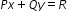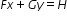# MAFS.912.A-REI.3.5Archived StandardExport Print
Prove that, given a system of two equations in two variables, replacing one equation by the sum of that equation and a multiple of the other produces a system with the same solutions.
General Information
Subject Area: Mathematics
Domain-Subdomain: Algebra: Reasoning with Equations & Inequalities
Cluster: Level 3: Strategic Thinking & Complex Reasoning
Cluster: Solve systems of equations. (Algebra 1 - Additional Cluster) (Algebra 2 - Additional Cluster) -

Clusters should not be sorted from Major to Supporting and then taught in that order. To do so would strip the coherence of the mathematical ideas and miss the opportunity to enhance the major work of the grade with the supporting clusters.

Date of Last Rating: 02/14
Status: State Board Approved - Archived
Assessed: Yes
Test Item Specifications
Assessed with:
MAFS.912.A-CED.1.2

Sample Test Items (1)
• Test Item #: Sample Item 1
• Question:

The systemhas the solution (3,-1) where F,G,H,P,Q and R are nonzero real numbers.Select all the systems that are also guaranteed to have the solution (3,-1).

• Difficulty: N/A
• Type: MS: Multiselect

## Related Courses

This benchmark is part of these courses.
1200310: Algebra 1 (Specifically in versions: 2014 - 2015, 2015 - 2022, 2022 and beyond (current))
1200320: Algebra 1 Honors (Specifically in versions: 2014 - 2015, 2015 - 2022, 2022 and beyond (current))
1200370: Algebra 1-A (Specifically in versions: 2014 - 2015, 2015 - 2022, 2022 and beyond (current))
1200400: Foundational Skills in Mathematics 9-12 (Specifically in versions: 2014 - 2015, 2015 - 2022, 2022 and beyond (current))
1200410: Mathematics for College Success (Specifically in versions: 2014 - 2015, 2015 - 2022 (course terminated))
1200700: Mathematics for College Algebra (Specifically in versions: 2014 - 2015, 2015 - 2022 (course terminated))
7912070: Access Mathematics for Liberal Arts (Specifically in versions: 2014 - 2015, 2015 - 2018, 2018 - 2019, 2019 - 2022, 2022 - 2023, 2023 and beyond (current))
7912080: Access Algebra 1A (Specifically in versions: 2014 - 2015, 2015 - 2018, 2018 - 2019, 2019 - 2022, 2022 and beyond (current))
1200315: Algebra 1 for Credit Recovery (Specifically in versions: 2014 - 2015, 2015 - 2022, 2022 and beyond (current))
1200375: Algebra 1-A for Credit Recovery (Specifically in versions: 2014 - 2015, 2015 - 2022, 2022 and beyond (current))
7912100: Fundamental Algebraic Skills (Specifically in versions: 2013 - 2015, 2015 - 2017 (course terminated))
1207300: Liberal Arts Mathematics 1 (Specifically in versions: 2014 - 2015, 2015 - 2022 (course terminated))
7912075: Access Algebra 1 (Specifically in versions: 2014 - 2015, 2015 - 2018, 2018 - 2019, 2019 - 2022, 2022 and beyond (current))

## Related Access Points

Alternate version of this benchmark for students with significant cognitive disabilities.

## Related Resources

Vetted resources educators can use to teach the concepts and skills in this benchmark.

## Formative Assessments

Solving Systems:

Students are given a system of two linear equations and asked to form a new system by replacing one equation with the sum of that equation and a multiple of the other. Then students are asked to explain why the two systems have the same solution.

Type: Formative Assessment

Solution Sets of Systems:

Students are asked to show that, given a system of two equations in two variables, replacing one equation with the sum of that equation and a multiple of another produces a system with the same solutions.

Type: Formative Assessment

## Lesson Plans

Take Me Out to the Ball Game!:

Students will research ticket sales at five different stadiums. They will then select two stadiums and develop a word problem. They will then solve to show that there is one solution and write and explanation why. This lesson is a project-based task that students can use to show their understanding of solving systems of equations.

Type: Lesson Plan

Changes are Coming! But Does it Really Matter? Exploring the Effects of “Special” Manipulations on the Solutions in a System of Equations.:

A great introductory lesson for solving systems of equations algebraically. Use as a follow up lesson to solving systems of equations graphically. Students will explore graphs of systems to see how manipulating the equations affects the solutions (if at all).

Type: Lesson Plan

Solving Linear Equations in Two Variables:

This lesson unit is intended to help you assess how well students are able to formulate and solve problems using algebra and, in particular, to identify and help students who have the following difficulties solving a problem using two linear equations with two variables and interpreting the meaning of algebraic expressions.

Type: Lesson Plan

## Perspectives Video: Professional/Enthusiast

Solving Systems of Equations, Oceans & Climate:

Angela Dial discusses how she solves systems of equations to determine how the composition of ocean floor sediment has changed over 65 million years to help reveal more information regarding climate change.

Type: Perspectives Video: Professional/Enthusiast

## Tutorials

Example 3: Solving Systems by Elimination:

This video is an example of solving a system of linear equations by elimination where the system has infinite solutions.

Type: Tutorial

Solving Systems of Linear Equations with Elimination Example 1:

This video shows how to solve a system of equations through simple elimination.

Type: Tutorial

Inconsistent Systems of Equations:

This video explains how to identify systems of equations without a solution.

Type: Tutorial

Example 2: Solving Systems by Elimination:

This video shows how to solve systems of equations by elimination.

Type: Tutorial

This video is an introduction to the elimination method of solving a system of equations.

Type: Tutorial

Trolls, tolls, and systems of equations:

This video tutorial discusses how to create a system of equations.

Type: Tutorial

## Unit/Lesson Sequence

Sample Algebra 1 Curriculum Plan Using CMAP:

This sample Algebra 1 CMAP is a fully customizable resource and curriculum-planning tool that provides a framework for the Algebra 1 Course. The units and standards are customizable and the CMAP allows instructors to add lessons, worksheets, and other resources as needed. This CMAP also includes rows that automatically filter and display Math Formative Assessments System tasks, E-Learning Original Student Tutorials and Perspectives Videos that are aligned to the standards, available on CPALMS.

Learn more about the sample Algebra 1 CMAP, its features and customizability by watching the following video:

### Using this CMAP

To view an introduction on the CMAP tool, please .

To view the CMAP, click on the "Open Resource Page" button above; be sure you are logged in to your iCPALMS account.

To use this CMAP, click on the "Clone" button once the CMAP opens in the "Open Resource Page." Once the CMAP is cloned, you will be able to see it as a class inside your iCPALMS My Planner (CMAPs) app.

To access your My Planner App and the cloned CMAP, click on the iCPALMS tab in the top menu.

All CMAP tutorials can be found within the iCPALMS Planner App or at the following URL: http://www.cpalms.org/support/tutorials_and_informational_videos.aspx

Type: Unit/Lesson Sequence

## Video/Audio/Animation

Why the Elimination Method Works:

This chapter presents a new look at the logic behind adding equations- the essential technique used when solving systems of equations by elimination.

Type: Video/Audio/Animation

## MFAS Formative Assessments

Solution Sets of Systems:

Students are asked to show that, given a system of two equations in two variables, replacing one equation with the sum of that equation and a multiple of another produces a system with the same solutions.

Solving Systems:

Students are given a system of two linear equations and asked to form a new system by replacing one equation with the sum of that equation and a multiple of the other. Then students are asked to explain why the two systems have the same solution.

## Student Resources

Vetted resources students can use to learn the concepts and skills in this benchmark.

## Tutorials

Example 3: Solving Systems by Elimination:

This video is an example of solving a system of linear equations by elimination where the system has infinite solutions.

Type: Tutorial

Solving Systems of Linear Equations with Elimination Example 1:

This video shows how to solve a system of equations through simple elimination.

Type: Tutorial

Inconsistent Systems of Equations:

This video explains how to identify systems of equations without a solution.

Type: Tutorial

Example 2: Solving Systems by Elimination:

This video shows how to solve systems of equations by elimination.

Type: Tutorial

This video is an introduction to the elimination method of solving a system of equations.

Type: Tutorial

Trolls, tolls, and systems of equations:

This video tutorial discusses how to create a system of equations.

Type: Tutorial

## Video/Audio/Animation

Why the Elimination Method Works:

This chapter presents a new look at the logic behind adding equations- the essential technique used when solving systems of equations by elimination.

Type: Video/Audio/Animation

## Parent Resources

Vetted resources caregivers can use to help students learn the concepts and skills in this benchmark.